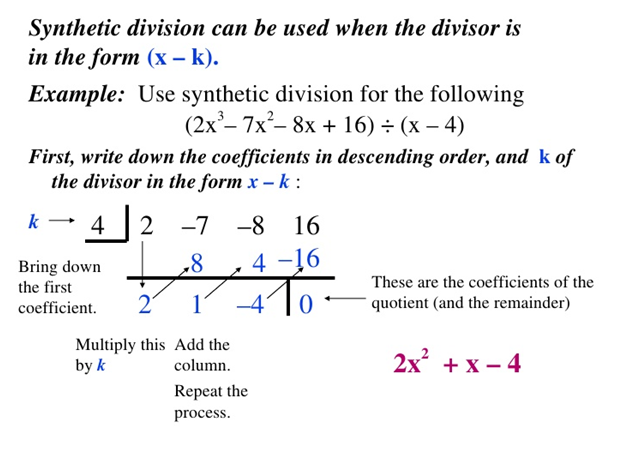# Section 4.2 - Polynomial Division

Section Objectives

1. Find the zeros of a polynomial and determine their multiplicities.
2. Carry out polynomial long division and synthetic division.
3. Apply the remainder and factor theorems.

### Polynomials

An $n$-th-degree polynomial in the variable $x$ is a function of the form

$f(x)=a_nx^n+a_{n-1}x^{n-1}+\cdots+a_1x+a_0$,

where the coefficients $a_n$, $a_{n-1}$, $\dots$, $a_0$ are real or complex numbers with $a_n \ne 0$.

The zeros of a function $f$ are those $x$-values for which $f(x)=0$. The real zeros of $f$ correspond to the $x$-intercepts of the graph of $f$: if the number $k$ is a zero of $f$, then $(k,0)$ is an $x$-intercept of the graph.

To find the zeros of a polynomial function, we must solve a polynomial equation of the form

$a_nx^n+a_{n-1}x^{n-1}+\cdots+a_1x+a_0=0.$

General polynomial equations are often solved by first making one side of the equation equal to zero. After doing so, the next steps may vary depending on the nature of the polynomial. For now, we will focus on solving by factoring.

### Factored polynomials and zeros

When a polynomial is completely factored, the number of times a specific linear factor occurs in the factorization is called the multiplicity of the corresponding zero.

#### Examples

• $g(x)=4(x-1)^3(x+5)(x+8)^2$ has zeros $x=1$, $x=-5$, and $x=-8$, with multiplicities 3, 1, and 2, respectively.

• Let $f(x)=x^7(x-1)^5(x+2)$. Find the zeros of $f$ and their multiplicities.

### Polynomial division

As long as you don't try to divide by zero, any two whole numbers can be divided to obtain a quotient and a remainder. For example, $26 \div 3 = 8 \mbox{ r } 3$.

In a similar way, a polynomial can be divided by a nonzero polynomial to form a quotient and remainder. In fact, given any polynomials $p(x)$ and $d(x) \ne 0$, there are unique polynomials $q(x)$ and $r(x)$ such that

where $r(x)=0$ or the degree of $r(x)$ is less than the degree of $d(x)$.

The quotient and remainder can be obtained by carrying out polynomial long division. In your end result, the degree of the remainder must be less than the degree of the divisor.

#### Examples

• Use long division to divide: $\quad \displaystyle \frac{3x^2+3x-14}{x-2}$

• Use long division to divide: $\quad (6x^3-5x^2-3) \div (3x+2)$

VERY IMPORTANT IDEA: When the polynomial $p(x)$ is divided by $(x-k)$, the remainder is the value $p(k)$. This idea often goes by the name of the Remainder Theorem.

#### Example

• Let $f(x)=2x^2+3x+1$. Use division to compute $f(2)$.

### Synthetic division

When a polynomial is divided by a 1st-degree polynomial of the form $(x-k)$, the long division process can be streamlined into a very efficient procedure called synthetic division.When using synthetic division, you must keep the following points in mind:

• Our form of synthetic division applies only when dividing by a polynomial of the form $(x-k)$, where $k$ can be any real or complex number.
• Be sure to write the coefficients of the dividend in order of descending exponents, including any missing zero coefficients.
• Be sure to use the correct sign of $k$.
• The number at the far right of the bottom row of your finished tableau is the remainder.

#### Examples

• Use synthetic division to divide: $\quad (x^3+3x^2-4x-12) \div (x+2)$

• Let $g(x)=x^3-5x+7$. Use synthetic division and the remainder theorem to compute $g(-3)$.

VERY IMPORTANT IDEA

Factor Theorem: $(x-k)$ is a factor of the polynomial $p(x)$ if and only if $k$ is a zero of $p(x)$.

#### Examples

• If a polynomial has zeros $x=0$, $x=3$, and $x=-1$, then it must have the corresponding factors $x$, $(x-3)$, and $(x+1)$.

• Determine if $(x-1)$ is a factor of $f(x)=7x^8+4x^5-9x^3-x^2-x$. Do you notice another factor?